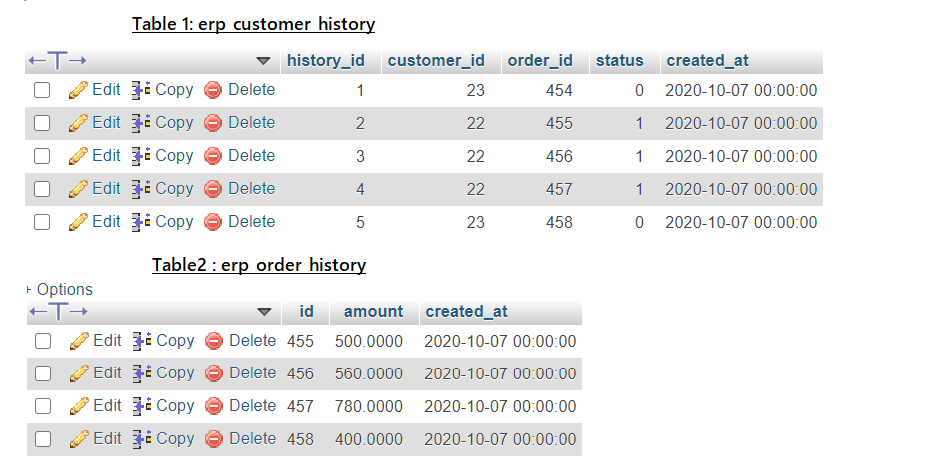# Magento 2 Direct SQL Queries in Zend FormatSometimes due to some project requirments, we need to directly communicate with Magento2 Database and we have to write SQL queries for that. This post will help you to write direct SQL Queries in Zend Format.

```public function __construct(
\Magento\Framework\App\ResourceConnection \$resourceConnection
) {
\$this->resourceConnection = \$resourceConnection;
}

```

Select Queries:

```\$connection = \$this->resourceConnection->getConnection();
\$tableName = \$this->resourceConnection->getTableName('customer_history');
\$tableName2 = \$this->resourceConnection->getTableName('order_history');```

Syntax:

```\$select = \$connection->select()
->from(
['p' => \$tableName])
->where('p.column_name=?', \$value)
->where('p.column_name2 >=?', \$value)
->order('p.column_name3 DESC')
->limit(\$pagesize, \$offset);

\$data = \$connection->fetchAll(\$select);```

Example:

```\$select = \$connection->select()
->from(
['p' => \$tableName])
->where('p.customer_id=?', 5)
->where('p.status =?', 1)
->order('p.createdat DESC')
->limit(10, 0);

\$data = \$connection->fetchAll(\$select);```

Perform Selet Query with JOIN

```\$select  = \$connection->select()
->from(
['p' => \$tableName], ['p.customer_id', 'p.status', 'p.order_id','o.ordertotal']
)
->join(
['o' => \$tableName2], 'main_table.order_id = order.id', ['']
)
->where('p.order_id = (?)', '455');

\$data = \$connection->fetchAll(\$query);```

Insert Query:

Syntax:

```\$data = ["column_name"=>\$value,"column_name2"=>\$value2,'column_name3'=>\$value3];

\$connection->insert(\$tableName, \$data);```

Example :

```\$data = ["customer_id"=>'22',"status"=>1,'order_id'=>'455'];

\$lastInsertedID= \$connection->insert(\$tableName, \$data);```

Update Query:

Syntax:

```\$data = ["column_name3"=>\$value3];

\$where = ['column_name = ?' => \$value, 'column_name2 = ?' => \$value2];

\$updatedRows=\$connection->update(\$tableName, \$data, \$where);```

Example:

```\$data = ["status"=>1];

\$where = ['customer_id = ?' => '22', 'order_id = ?' => '455'];

\$updatedRows=\$connection->update(\$tableName, \$data, \$where);```

Delete Queries

Syntax:

```\$connection->delete(
\$tableName,
['column_name = ?' => \$value, 'column_name2 = ?' => '0']
);```

Example :

```\$connection->delete(
\$tableName,
['customer_id = ?' => '22', 'status = ?' => '0']
);```

1   0